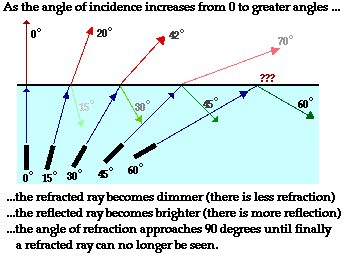When a beam of light passes through denser medium to a rarer medium it curves away from the normal at the point that divides the two medium. This happens due to refraction.

The ray of light keeps moving away from the normal as the angle of incidence (θi) and the angle of refraction (θr) increases simultaneously. After some time, the angle of refraction will reach 90 degree for certain value of angle of incidence. This value of angle of incidence for which the angle of refraction reaches 90 degree is called the critical angle.A total internal reflection occurs when the refracted ray gets constricted to the same medium. This happens when the angle of incidence becomes greater than the critical angle which is 90 degree. When this happens the angle of refraction gets greater than 90 degree.